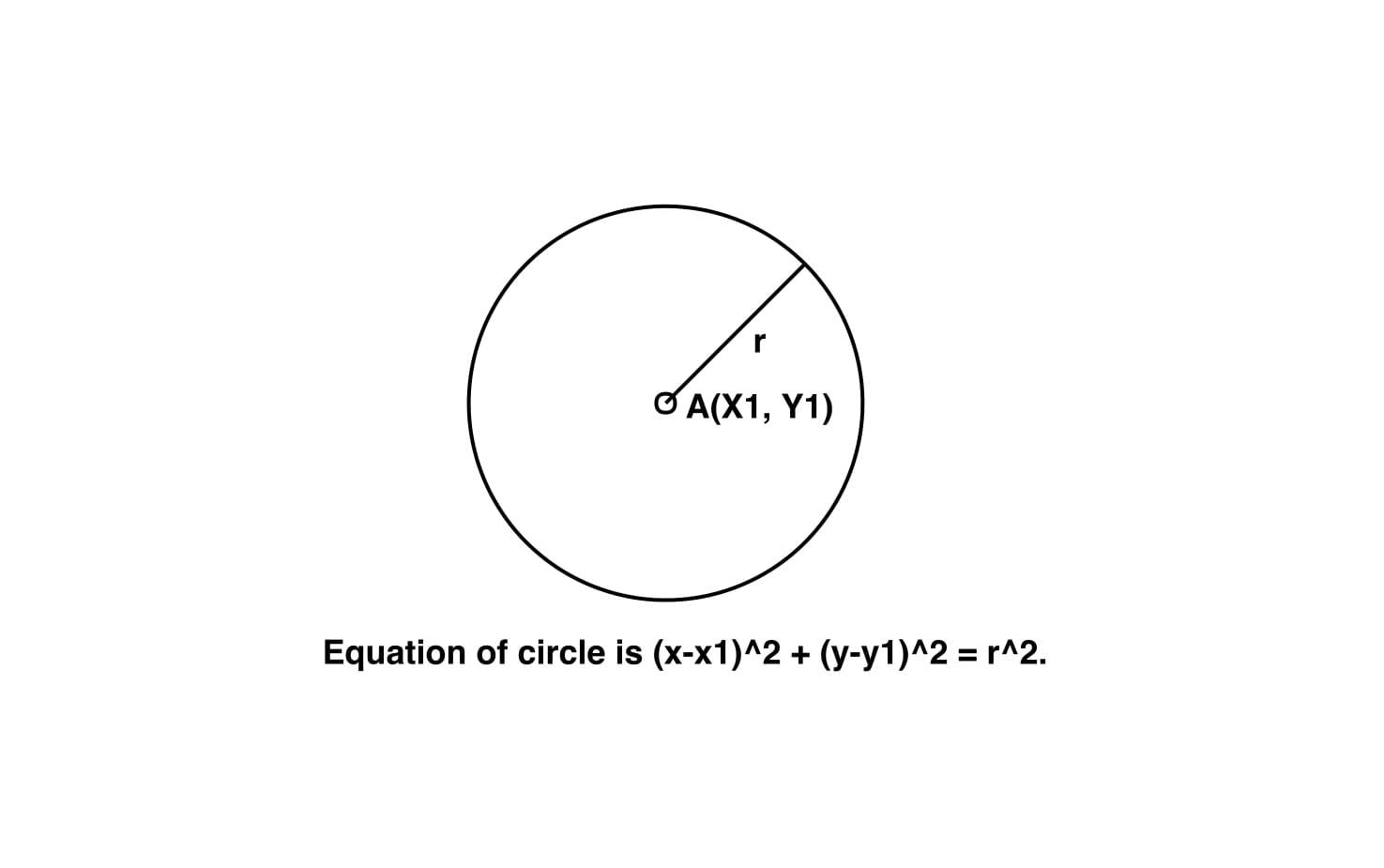# Equation of circle from centre and radius

Given the centre of circle (x1, y1) and its radius r, find the equation of the circle having centre (x1, y1) and having radius r.

Examples:

Input : x1 = 2, y1 = -3, r = 8
Output : x^2 + y^2 – 4*x + 6*y = 51.

Input : x1 = 0, y1 = 0, r = 2
Output : x^2 + y^2 – 0*x + 0*y = 4.

## Recommended: Please try your approach on {IDE} first, before moving on to the solution.Approach:
Given the centre of circle (x1, y1) and its radius r, we have to find the equation of the circle having centre (x1, y1) and having radius r.
the equation of circle having centre (x1, y1) and having radius r is given by :-

``

on expanding above equation

``

on arranging above we get

``

Below is the implementation of above approach:

## C++

 `// CPP program to find the equation ` `// of circle. ` `#include ` `using` `namespace` `std; ` ` `  `// Function to find the equation of circle ` `void` `circle_equation(``double` `x1, ``double` `y1, ``double` `r) ` `{ ` `    ``double` `a = -2 * x1; ` ` `  `    ``double` `b = -2 * y1; ` ` `  `    ``double` `c = (r * r) - (x1 * x1) - (y1 * y1); ` ` `  `    ``// Printing result ` `    ``cout << ``"x^2 + ("` `<< a << ``" x) + "``; ` `    ``cout << ``"y^2 + ("` `<< b << ``" y) = "``; ` `    ``cout << c << ``"."` `<< endl; ` `} ` ` `  `// Driver code ` `int` `main() ` `{ ` `    ``double` `x1 = 2, y1 = -3, r = 8; ` `    ``circle_equation(x1, y1, r); ` `    ``return` `0; ` `} `

## Java

 `// Java program to find the equation ` `// of circle. ` `import` `java.util.*; ` ` `  `class` `solution ` `{ ` ` `  ` ``// Function to find the equation of circle ` `static` `void` `circle_equation(``double` `x1, ``double` `y1, ``double` `r) ` `{ ` `    ``double` `a = -``2` `* x1; ` ` `  `    ``double` `b = -``2` `* y1; ` ` `  `    ``double` `c = (r * r) - (x1 * x1) - (y1 * y1); ` ` `  `    ``// Printing result ` `    ``System.out.print(``"x^2 + ("` `+a+ ``" x) + "``); ` `     ``System.out.print(``"y^2 + ("``+b + ``" y) = "``); ` `     ``System.out.println(c +``"."` `); ` `} ` ` `  `// Driver code ` `public` `static` `void` `main(String arr[]) ` `{ ` `    ``double` `x1 = ``2``, y1 = -``3``, r = ``8``; ` `    ``circle_equation(x1, y1, r); ` `  `  `} ` ` `  `} `

## Python3

 `# Python3 program to find the  ` `# equation of circle. ` ` `  `# Function to find the  ` `# equation of circle ` `def` `circle_equation(x1, y1, r): ` `    ``a ``=` `-``2` `*` `x1; ` ` `  `    ``b ``=` `-``2` `*` `y1; ` ` `  `    ``c ``=` `(r ``*` `r) ``-` `(x1 ``*` `x1) ``-` `(y1 ``*` `y1); ` ` `  `    ``# Printing result ` `    ``print``(``"x^2 + ("``, a, ``"x) + "``, end ``=` `""); ` `    ``print``(``"y^2 + ("``, b, ``"y) = "``, end ``=` `""); ` `    ``print``(c, ``"."``); ` ` `  `# Driver code ` `x1 ``=` `2``;  ` `y1 ``=` `-``3``;  ` `r ``=` `8``; ` `circle_equation(x1, y1, r); ` ` `  `# This code is contributed  ` `# by mits `

## C#

 `// C# program to find the equation ` `// of circle. ` `using` `System; ` ` `  `class` `GFG ` `{ ` ` `  `// Function to find the equation of circle ` `public` `static` `void` `circle_equation(``double` `x1, ` `                                   ``double` `y1,  ` `                                   ``double` `r) ` `{ ` `    ``double` `a = -2 * x1; ` ` `  `    ``double` `b = -2 * y1; ` ` `  `    ``double` `c = (r * r) - (x1 * x1) - (y1 * y1); ` ` `  `    ``// Printing result ` `    ``Console.Write(``"x^2 + ("` `+ a + ``" x) + "``); ` `    ``Console.Write(``"y^2 + ("``+ b + ``" y) = "``); ` `    ``Console.WriteLine(c + ``"."` `); ` `} ` ` `  `// Driver code ` `public` `static` `void` `Main(``string` `[]arr) ` `{ ` `    ``double` `x1 = 2, y1 = -3, r = 8; ` `    ``circle_equation(x1, y1, r); ` `} ` `} ` ` `  `// This code is contributed  ` `// by SoumkMondal `

## PHP

 ` `

Output:

```x^2 + (-4 x) + y^2 + (6 y) = 51.
```

My Personal Notes arrow_drop_upCheck out this Author's contributed articles.

If you like GeeksforGeeks and would like to contribute, you can also write an article using contribute.geeksforgeeks.org or mail your article to contribute@geeksforgeeks.org. See your article appearing on the GeeksforGeeks main page and help other Geeks.

Please Improve this article if you find anything incorrect by clicking on the "Improve Article" button below.